# Python | Pandas Index.append()

• Last Updated : 16 Dec, 2018

Python is a great language for doing data analysis, primarily because of the fantastic ecosystem of data-centric python packages. Pandas is one of those packages and makes importing and analyzing data much easier.

Pandas` Index.append()` function is used to append a single or a collection of indices together. In case of collection of indices, all of them gets appended to the original index in the same order as they are passed to the `Index.append()` function. The function returns an appended index.

Syntax: Index.append(other)

Parameters :
other : Index or list/tuple of indices

Returns : appended : bool or array_like (if axis is specified)
A single element array_like may be converted to bool.

Example #1: Use `Index.append()` function to append a single index to the given index.

 `# importing pandas as pd``import` `pandas as pd`` ` `# Creating the first Index``df1 ``=` `pd.Index([``17``, ``69``, ``33``, ``5``, ``0``, ``74``, ``0``])`` ` `# Creating the second Index``df2 ``=` `pd.Index([``11``, ``16``, ``54``, ``58``])`` ` `# Print the first and second Index``print``(df1, ``"\n"``, df2)`

Output :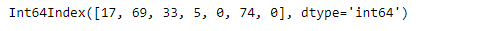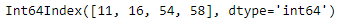Let’s append df2 index at the end of df1.

 `# append df2 at the end of df1``df1.append(df2)`

Output :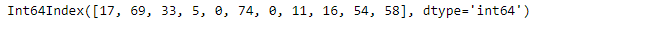As we can see in the output, the second index i.e. df2 has been appended at the end of df1.

Example #2: Use `Index.append()` function to append a collection of index at the end of the given index.

 `# importing pandas as pd``import` `pandas as pd`` ` `# Creating the first Index``df1 ``=` `pd.Index([``'Jan'``, ``'Feb'``, ``'Mar'``, ``'Apr'``])`` ` `# Creating the second Index``df2 ``=` `pd.Index([``'May'``, ``'Jun'``, ``'Jul'``, ``'Aug'``])`` ` `# Creating the third Index``df3 ``=` `pd.Index([``'Sep'``, ``'Oct'``, ``'Nov'``, ``'Dec'``])`` ` `# Print the first, second and third Index``print``(df1, ``"\n"``, df2, ``"\n"``, df3)`

Output :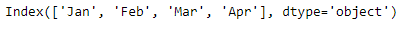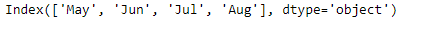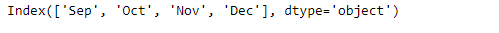Let’s append both the indexes df2 and df3 at the end of df1.

 `# We pass df2 and df3 as a list of``# indexes to the append function``df1.append([df2, df3])`

Output :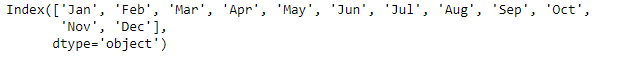My Personal Notes arrow_drop_up